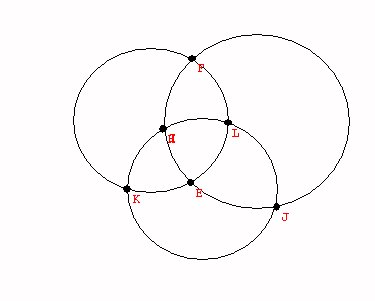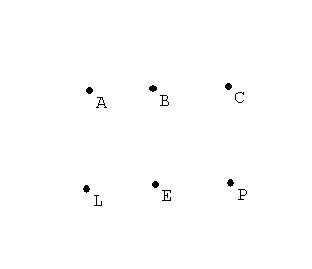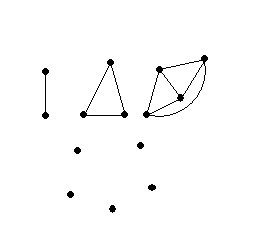Thurrsday,  March 2
• Topology and measurements:

• Counting on a line.  Counting on a curve.
Keep count on the number of vertices and segments for a graph.   Compare:

 Vertices Edges V-E Line segment I 2 1 1 circle O 1 1 0 8 1 2 -1 9 2 2 0 B 2 3 -1

Curves will not be topologically equivalent if the number V-E is not the same.

However, as the table shows, there are curves that have the same number V-E which are also not equivalent.

These can be distinguished by other criterion, such as connectivity when a point is removed.

The
number V-E is a characteristic of the curve
that will be the same for any topologically equivalent curve.

However, this number does not completely classify the curves topologically, since curves can have the same number V-E without being equivalent.

Review from last class:

1. Counting in the plane or Counting on a surface.
Vertices, edges, regions.

 Vertices Regions Edges Line segment 2 1 1 circle 1 2 1 8 1 3 2 9 2 2 2 B 2 3 36 8 12

Counting in the plane or Counting on a sphere.

Euler's Formula for the plane or the sphere:
Theorem: For any connected graph in the plane,

V+R = E + 2.
OR  V -E+R =2

The number 2 is called the "Euler characteristic for the plane."  ( and the sphere).

The idea of the Proof:  Deconstruct the graph.
The relation between V+R and E+ 2 stays the same.  .... [First to obtain one region, then to obtain a single vertex... staying connected at all times!]
Finally we have just one vertex, no edges, and one region.
So the relationship is that V+R = E + 2!

For any connected graph on the sphere, V+R = E + 2

Proof:
Choose a point P on the sphere not on the graph.
Place a plane touching the sphere opposite to P.
Then project the graph onto the plane.

The projected graph will have the same number of vertices, edges and regions.

The counting in the plane shows that the formula is true for the projected graph, and thus for the original graph on the sphere!

1. What about graphs on the Torus?
We have a connected graph on the torus with one vertex, two edges and only on region. But this information does not match for the euler formula for the plane or the sphere. SO.. the torus must be topologically different from the sphere or the plane!
In fact for the torus we see that it is possible for V+R= E   or  V-E+R= 0

Summary: We have learned about (and proved) Euler's Formula for the plane or the sphere:

Theorem: For any connected graph in the plane,
V+R = E + 2.
OR  V -E+R =2

The number 2 is called the "Euler characteristic for the plane."  ( and the sphere).

This formula can help solve some problems on the plane and elsewhere.
We'll continue to examine more applications of this topological property of graphs (networks) in the plane and eventually use it to prove a result about coloring!

The Utilities problem:
There are three houses being planned on a new development owned by Aaron(A), Beth(B), and Carol (C).

The phone company(P), cable company(L), and electric company(E) are planning to put in underground lines to the three houses and to minimize interference between the services, they would like to set up separate lines from there connection boxes to the houses that do not cross on the map of the development.
Draw a design that will achieve this objective.\$10 reward to any and all who solve the problem!

Discussion: Analysis. Suppose this were possible and consider the consequences.
If the graph were drawn it would have V = 6 vertices and E=?__   _   edges.
Based on Euler's formula, the graph would therefore have to have  R=?_   __ regions in the plane.

By design each region would have to have at least 4 edges.
Thus if we count the edges by the regions we would have at least 4R edges counting each edge twice- because an edge is a boundary for exactly two regions.
Thus the number of edges must be more than 2R.
Is this possible? What can we conclude?

Complete graphs. A complete graph is a graph in which each vertex of the graph is connected by an edge to every other vertex.

For example:

2 vertices: 1 edge - a line segment
3 vertices: 3 edges -  a triangle
4 vertices: 6  edges - a tetrahedron
Notice these all have planar representations.
The technical way this is decribed is to say these graphs can be imbedded in the plane.
Draw a complete graph using 5 vertices.Discussion: Analysis. Suppose this were possible and consider the consequences.
If the graph were drawn it would have V = 5 vertices and E= ?_    __ edges.
Based on Euler's formula, the graph would therefore have to have R=?                regions in the plane.

By design each region would have to have at least 3 edges.
Thus if we count the edges by the regions we would have at least 3R=21 edges counting each edge twice- because an edge is a boundary for exactly two regions.
Thus the number of edges must be more than 3/2R=10 1/2.
Is this possible? What can we conclude?

Coloring problems: Suppose we have a map that consists of a graph that determines R regions (countries) with E edges (borders).  How many colors are needed to color the regions so that countries that share a border (not merely a vertex) have different colors.
The Junkyard on the color problem.